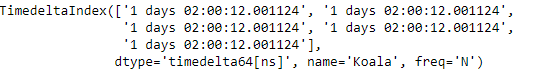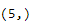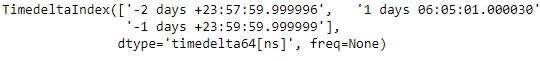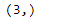Related Articles
Python | Pandas TimedeltaIndex.shape
• Last Updated : 29 Dec, 2018

Python is a great language for doing data analysis, primarily because of the fantastic ecosystem of data-centric python packages. Pandas is one of those packages and makes importing and analyzing data much easier.

Pandas` TimedeltaIndex.shape` attribute return a tuple of the shape of the underlying data present in the object under consideration.

Syntax : TimedeltaIndex.shape

Return : shape

Example #1: Use `TimedeltaIndex.shape` attribute to find out the shape of the underlying data in the given object.

 `# importing pandas as pd``import` `pandas as pd`` ` `# Create the TimedeltaIndex object``tidx ``=` `pd.TimedeltaIndex(start ``=``'1 days 02:00:12.001124'``, periods ``=` `5``,``                                             ``freq ``=``'N'``, name ``=``'Koala'``)`` ` `# Print the TimedeltaIndex``print``(tidx)`

Output :Now we will find out the shape of the given object.

 `# print the shape of the tidx object``tidx.shape`

Output :As we can see in the output, the `TimedeltaIndex.shape` attribute has returned the (5, ) which is the shape of the underlying data of the tidx object.

Example #2: Use `TimedeltaIndex.shape` attribute to find out the shape of the underlying data in the given object.

 `# importing pandas as pd``import` `pandas as pd`` ` `# Create the TimedeltaIndex object``tidx ``=` `pd.TimedeltaIndex(data ``=``[``'-1 days 2 min 3us 10ns'``, ``'1 days 06:05:01.000030'``,``                                                      ``'-1 days + 23:59:59.999999'``])`` ` `# Print the TimedeltaIndex``print``(tidx)`

Output :Now we will find out the shape of the given object.

 `# print the shape of the tidx object``tidx.shape`

Output :As we can see in the output, the `TimedeltaIndex.shape` attribute has returned the (3, ) which is the shape of the underlying data of the tidx object.

Attention geek! Strengthen your foundations with the Python Programming Foundation Course and learn the basics.

To begin with, your interview preparations Enhance your Data Structures concepts with the Python DS Course.

My Personal Notes arrow_drop_up